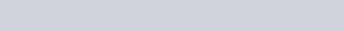# Python Modulo

Filed Under: Python

Good day, learners. In our previous tutorial, we learned about Python NumPy Module. In this tutorial we are going to see examples of Python Modulo.

## Python Modulo

Basically Python modulo operation is used to get the reminder of a division. The basic syntax of Python Modulo is `a % b`. Here `a` is divided by `b` and the remainder of that division is returned.

In many language, both operand of this modulo operator has to be integer. But Python Modulo is flexible in this case. The operands can be either `integer` or `float`.

### Python Modulo Example

We have discussed a little bit about modulo operation in the python operators tutorial. Now we will see python modulo example here.

In the following program you will be asked if you want to continue the program. By pressing ‘y’ means, you want to continue. Otherwise, the program will terminate instantly.

When you continue with the program, you have to enter two numbers and by using modulo operation, the reminder will be printed.

``````
while True:
a = input('Do you want to continue? ')
if a.lower() != 'y':
break

a = float(input('Enter a number : '))
b = float(input('Enter another number : '))
print(a, ' % ', b, ' = ', a % b)
print(b, ' % ', a, ' = ', b % a)

``````

The output will vary for different input. For my input, the output was the following:

``````
Do you want to continue? y
Enter a number : 12
Enter another number : 3
12.0  %  3.0  =  0.0
3.0  %  12.0  =  3.0
Do you want to continue? y
Enter a number : 2
Enter another number : 3
2.0  %  3.0  =  2.0
3.0  %  2.0  =  1.0
Do you want to continue? y
Enter a number : 1.2
Enter another number : 2.1
1.2  %  2.1  =  1.2
2.1  %  1.2  =  0.9000000000000001
Do you want to continue? n
``````

### Python Modulo Operator Exception

The only Exception you get with python modulo operation is `ZeroDivisionError` error. This happens if the divider operand of the modulo operator become zero. That means the right operand can’t be zero. Let’s see the following code to know about this python exception.

``````
a = 12
b = 0
try:
print(a, ' % ', b, ' = ', a % b)
except ZeroDivisionError as z:
print('Cannot divide by zero! :) ')
``````

The output of the following code will be like thisThat’s all about python modulo example. If you have any query feel free to use the comment box.

Reference: Official Documentation, ZeroDivisionError

1.Sadula subba reddy says: